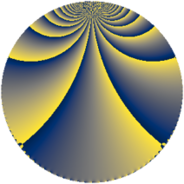# Properties

 Label 2736.3.bjLevel $2736$ Weight $3$ Character orbit 2736.bj Rep. character $\chi_{2736}(1247,\cdot)$ Character field $\Q(\zeta_{6})$ Dimension $480$ Sturm bound $1440$

# Related objects

## Defining parameters

 Level: $$N$$ $$=$$ $$2736 = 2^{4} \cdot 3^{2} \cdot 19$$ Weight: $$k$$ $$=$$ $$3$$ Character orbit: $$[\chi]$$ $$=$$ 2736.bj (of order $$6$$ and degree $$2$$) Character conductor: $$\operatorname{cond}(\chi)$$ $$=$$ $$684$$ Character field: $$\Q(\zeta_{6})$$ Sturm bound: $$1440$$

## Dimensions

The following table gives the dimensions of various subspaces of $$M_{3}(2736, [\chi])$$.

Total New Old
Modular forms 1944 480 1464
Cusp forms 1896 480 1416
Eisenstein series 48 0 48

## Trace form

 $$480q + 12q^{9} + O(q^{10})$$ $$480q + 12q^{9} - 216q^{17} - 2400q^{25} + 180q^{33} + 216q^{41} + 1680q^{49} + 60q^{57} - 432q^{65} + 36q^{73} - 564q^{81} - 540q^{97} + O(q^{100})$$

## Decomposition of $$S_{3}^{\mathrm{new}}(2736, [\chi])$$ into newform subspaces

The newforms in this space have not yet been added to the LMFDB.

## Decomposition of $$S_{3}^{\mathrm{old}}(2736, [\chi])$$ into lower level spaces

$$S_{3}^{\mathrm{old}}(2736, [\chi]) \cong$$ $$S_{3}^{\mathrm{new}}(684, [\chi])$$$$^{\oplus 3}$$